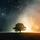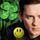## General Question# How do I calculate average annual rate of return?

Asked by 2davidc8 (10183) May 4th, 2017

Suppose I bought something (say, a house) for \$53,000 and it’s worth \$650,000 40 years later. What’s the average rate of return?

I used to know how to calculate this. What’s the formula?

(And, no, this is not a homework question. I’ve not been in school in decades.)

Observing members: 0Composing members: 0You want to find the solution of 53,000*x**40 = 650,000, Divide 650,000 by 53,000 and then take the 40th root of that. 40th root is the same as raising to the power or ¼0 = .025. I get a little under 1.065, which means a 6.5% average growth rate.

LostInParadise (30445)“Great Answer” (2) Flag as…^ That is the best answer. It gives the exact figure. Here is a way to estimate it in your head.

Round off the numbers for easy math. 53 becomes 50 and 650 becomes 600.
Your value increased by 600/50 = 12 times.
The Rule of 70 says the interest rate x the doubling period is 70 Examples: An investment getting 7% will double in 10 years (70/7). An investment getting 10% will double in 7 years. An investment that doubles in 2 years is getting 35%. Got it? Now let’s use it.
You had your house for 40 years . That is four 10 year periods. If the value increased times 2×2 x 2×2 \$800k the rate would be 7%. But your value only increased 12 times so the interest rate is a little less than than that. Try 6%. That would double every ~12 years which results in only 3+ a little doubles 2×2 x 2 = 8 x 50k = 400 which is much too low. I’d go halfway in-between the 400k number and the 800k number to get 6.5% over the 40 year period.

This is a good way to sense check your exact calculation.

LuckyGuy (41355)“Great Answer” (1) Flag as…I vaguely recall that method. I was curious as to why it works and am somewhat embarrassed to say that I couldn’t figure it out right away. I did a Web search and found this simple explanation. It all boils down to the facts that you can use natural logarithms to solve problems involving continuous interest and that ln(2) = .693, which is close to .70. You get from decimal representation of an interest rate to percent by multiplying by 100 and if you also multiply .693 by 100, you get 69.3, which is close to 70. Follow the link for the details.

Continuous interest is not the same as interest compounded yearly, which is what I originally computed. For most calculations the difference is relatively minor. Using the formula in the link to compute what the continuous interest rate would be, we get
650,000 = 53,000 e**(40r).
650/53 = e**(40r).
Taking the natural log of both sides gives 40r = ln(650/53). Performing the calculation on the right and dividing by 40 gives about 6.3%.

LostInParadise (30445)“Great Answer” (1) Flag as…WOW, thanks, jellies! It’s a bit more complicated than I remembered. Much appreciated!

2davidc8 (10183)“Great Answer” (0) Flag as…or